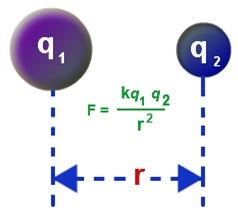# Coulombs Law Formula

## Coulomb’s Law Formula

Coulomb’s law is based on the electrostatic interaction between electrically charged particles. The law was first published by French physicist Charles Augustin de Coulomb and was essential in the development of the theory of electromagnetism.

The law provides the amount of force when two charges are separated by some distance.

The interaction among charged objects is a non-contact force that acts at some distance of separation. Every electrical interaction involves a force that highlights the importance of charged variables.

Coulomb’s law on magnitude states that,

The electrostatic force between the two charged points is directly proportional to the product of these charges and inversely proportional to the square of the distance between them.Coulomb’s Law Formula is expressed as

Wherein,

q1 =magnitude of first charge

q2 = magnitude of the second charge

r = distance between the two charges

k = Coulomb Constant ( 9 × 10-9 N m2/C)

Coulomb’s law formula is applied to calculate the force exerted on the charge and distance if these quantities are given.

The unit of Coulomb force is expressed in Newton (N).

Example1

If the force between the two charges 5 μ C and 9 μ C is .05 N, Calculate the distance by which they are separated?

Solution:

Given: Charge q1 = 5 μ C,

Charge q2 = 9 μ C,

Force F = 0.05 N

Electric force constant k = 9 × 10-9 Nm2/C2

The force formula is given by F = q1q2 / r2

Distance between them is given by r = q1q2 / F

Distance r = 9×10-9 × 5 ×10−6 x 9×10−6 / 0.05

= 8.1 m.

Example 2

If four protons surround an electron, and they are separated by 2 μ m, determine the Electrostatic force acting between them.

Charge of proton = 1.6 × 10-19 C

Charge of electron = – 1.6 × 10-19 C

Solution

Given:

Charge q1 = 4 protons

= 4 × 1.6 ×10-19 C = 6.4 × 10-19 C

Charge q2 = 1 electron

= – 1.6 x 10-19 C

Electrostatic force formula is given by

F = k q1q2 / r2

= 9×10-9 × 6.4×10−19×−1.6×10−19 / (2×10−6)2

= – 2.3 ×10-16 N

#### Practise This Question

State whether true or false- Two equivalent fractions can never be like fractions.